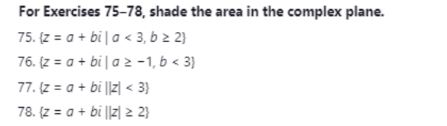# For Exercises 75-78, shade the area in the complex plane.75. (z = a + bila < 3, b 2 2)76. (z = a + bi |a 2-1, 6 < 3)77. (z = a + bi ||z| < 3}78. (z = a + bi ||z| 2 2}

Question
1 viewshelp_outlineImage TranscriptioncloseFor Exercises 75-78, shade the area in the complex plane. 75. (z = a + bila < 3, b 2 2) 76. (z = a + bi |a 2-1, 6 < 3) 77. (z = a + bi ||z| < 3} 78. (z = a + bi ||z| 2 2} fullscreen
check_circle

Step 1

We’ll answer the first question since the exact one wasn’t specified. Please submit a new question specifying the one you’d like answered.

#75) Given {Z=a+ib | a<3 , b≥2}

Let x+iy is any point in the set.

So we get x<3 and y≥2

Step 2

We draw x=3 and y=-2...

### Want to see the full answer?

See Solution

#### Want to see this answer and more?

Solutions are written by subject experts who are available 24/7. Questions are typically answered within 1 hour.*

See Solution
*Response times may vary by subject and question.
Tagged in

### Other